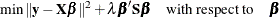# The GAMPL Procedure

### Thin-Plate Regression Splines

Subsections:

The GAMPL procedure uses thin-plate regression splines (Wood 2003) to construct spline basis expansions. The thin-plate regression splines are based on thin-plate smoothing splines (Duchon 1976, 1977). Compared to thin-plate smoothing splines, thin-plate regression splines produce fewer basis expansions and thus make direct fitting of generalized additive models possible.

#### Thin-Plate Smoothing Splines

Consider the problem of estimating a smoothing function f ofwith d covariates from n observations. The model assumes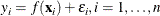Then the thin-plate smoothing splines estimate the smoothing function f by minimizing the penalized least squares function: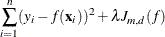The penalty term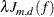includes the function that measures roughness on the f estimate: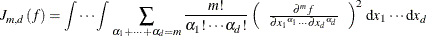The parameter m (which corresponds to the M= option for a spline effect) specifies how the penalty is applied to the function roughness. Function derivatives whose order is less than m are not penalized. The relation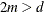must be satisfied.

The penalty term also includes the smoothing parameter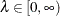, which controls the trade-off between the model’s fidelity to the data and the function smoothness of f. When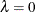, the function estimate corresponds to an interpolation. When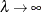, the function estimate becomes the least squares fit. By using the defined penalized least squares criterion and a fixed
value, you can explicitly express the estimate of the smooth function f in the following form: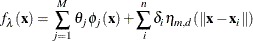In the expression of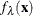,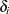and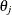are coefficients to be estimated. The functions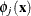correspond to unpenalized polynomials ofwith degrees up to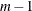. The total number of these polynomials is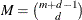. The function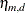models the extra nonlinearity besides the polynomials and is a function of the Euclidean distance r between anyvalue and an observed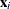value: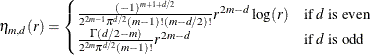Define the penalty matrix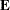such that each entry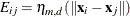, letbe the vector of the response, let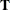be the matrix where each row is formed by, and let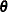and
be vectors of coefficientsand. Then you can obtain the function estimate
from the following minimization problem: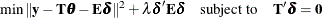For more information about thin-plate smoothing splines, see Chapter 116: The TPSPLINE Procedure in SAS/STAT 14.1 User's Guide.

#### Low-Rank Approximation

Given the representation of the thin-plate smoothing spline, the estimate of f involves as many parameters as the number of unique data points. Solving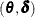with an optimumbecomes difficult for large problems.

Because the matrixis symmetric and nonnegative definite, the eigendecomposition can be taken as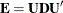, where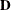is the diagonal matrix of eigenvalues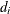of, and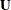is the matrix of eigenvectors that corresponds to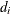. The truncated eigendecomposition forms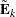, which is an approximation tosuch that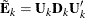where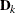is a diagonal matrix that contains the k most extreme eigenvalues in descending order of absolute values: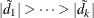.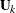is the matrix that is formed by columns of eigenvectors that correspond to the eigenvalues in.

The approximationnot only reduces the dimension fromofto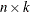but also is optimal in two senses. First,minimizes the spectral norm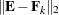betweenand all rank k matrices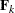. Second,also minimizes the worst possible change that is introduced by the eigenspace truncation as defined by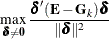where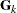is formed by any k eigenvalues and corresponding eigenvectors. For more information, see Wood (2003).

Now given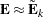and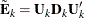, and letting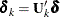, the minimization problem becomes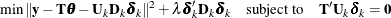You can turn the constrained optimization problem into an unconstrained one by using any orthogonal column basis. One way to formis via the QR decomposition of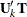: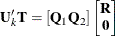Let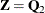. Then it is verified that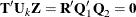So for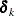such that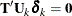, it is true that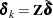. Now the problem becomes the unconstrained optimization,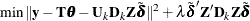Let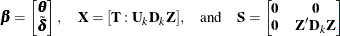The optimization is simplified as